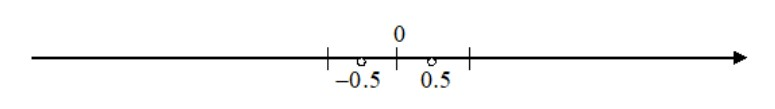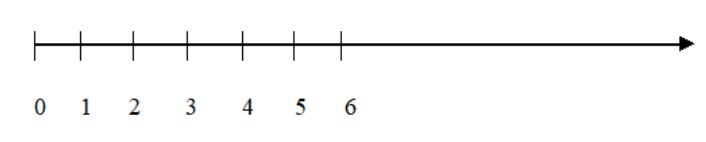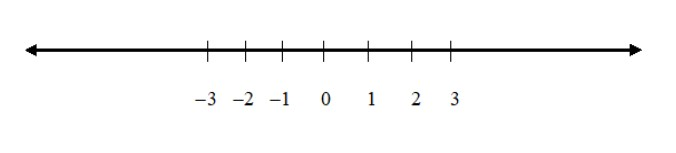QuestionAnswers

# Write a rational number and its opposite in the decimal form. How do you know that these are opposite rational numbers? Write a rational number and its opposite in the fraction form.

Hint:Rational numbers are the numbers which can be expressed as $\dfrac{p}{q}$ ratio, where $p$, $q$ are integers and $q \ne 0$. The opposite of a number is the same distance from 0 as the number itself, but on the other side of 0 on a number line.

We need to first write a rational number.
Also we have to find its opposite in the decimal form.
Let us the take the rational number $\dfrac{1}{2}$ (It is a rational number because we can expressed it as $\dfrac{p}{q}$ ratio, where $p$, $q$ are integers and $q \ne 0$).
The decimal form of this number is $0.5$ .
It’s opposite in the decimal form is $- 0.5$.In the diagram, $0.5$ is the opposite of $- 0.5$, and $- 0.5$ is the opposite of $0.5$. The distance from $0.5$ to $0$ is $0.5$, and the distance from$- 0.5$ to $0$ is $0.5$; this distance to $0$ is the same for both $0.5$ and $- 0.5$. The absolute value of a number is its distance from $0$ on a number line.
Let us take the rational number $\dfrac{3}{5}$ , it’s opposite in the fraction form is $- \dfrac{3}{5}$.

Note:
Whole numbers: Whole numbers are simply the numbers $0,\;{\text{ }}1,{\text{ }}2,\,{\text{ }}3,{\text{ }}4,{\text{ }}5,\,{\text{ }}6,\;....$Integers: Integers are like whole numbers, but they also include negative numbers but still no fractions allowed.So integers can be negative {$- 1, - 2, - 3, - 4, - 5, - 6,....$}, positive {$1,2,3,4,5,6,....$} or zero {$0$}.
Rational number:Rational numbers are the numbers which can be expressed as $\dfrac{p}{q}$ ratio, where $p$, $q$ are integers and $q \ne 0$.